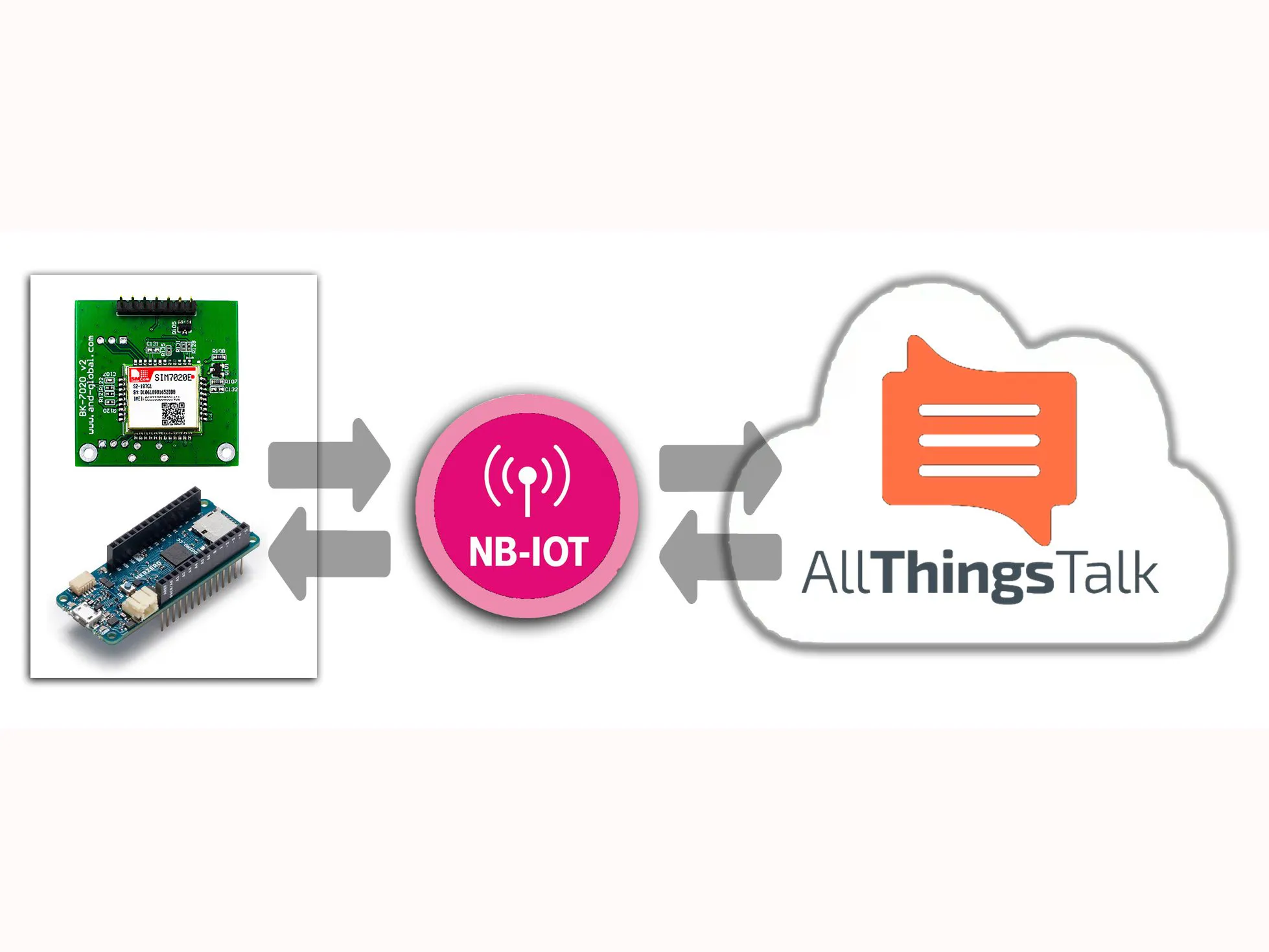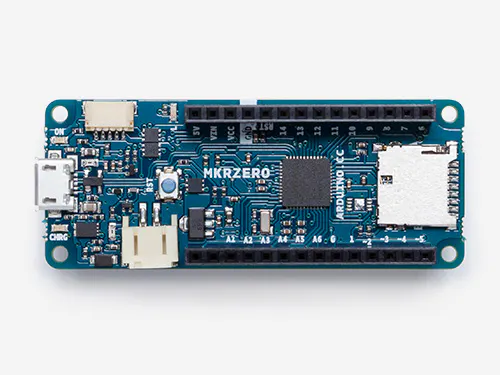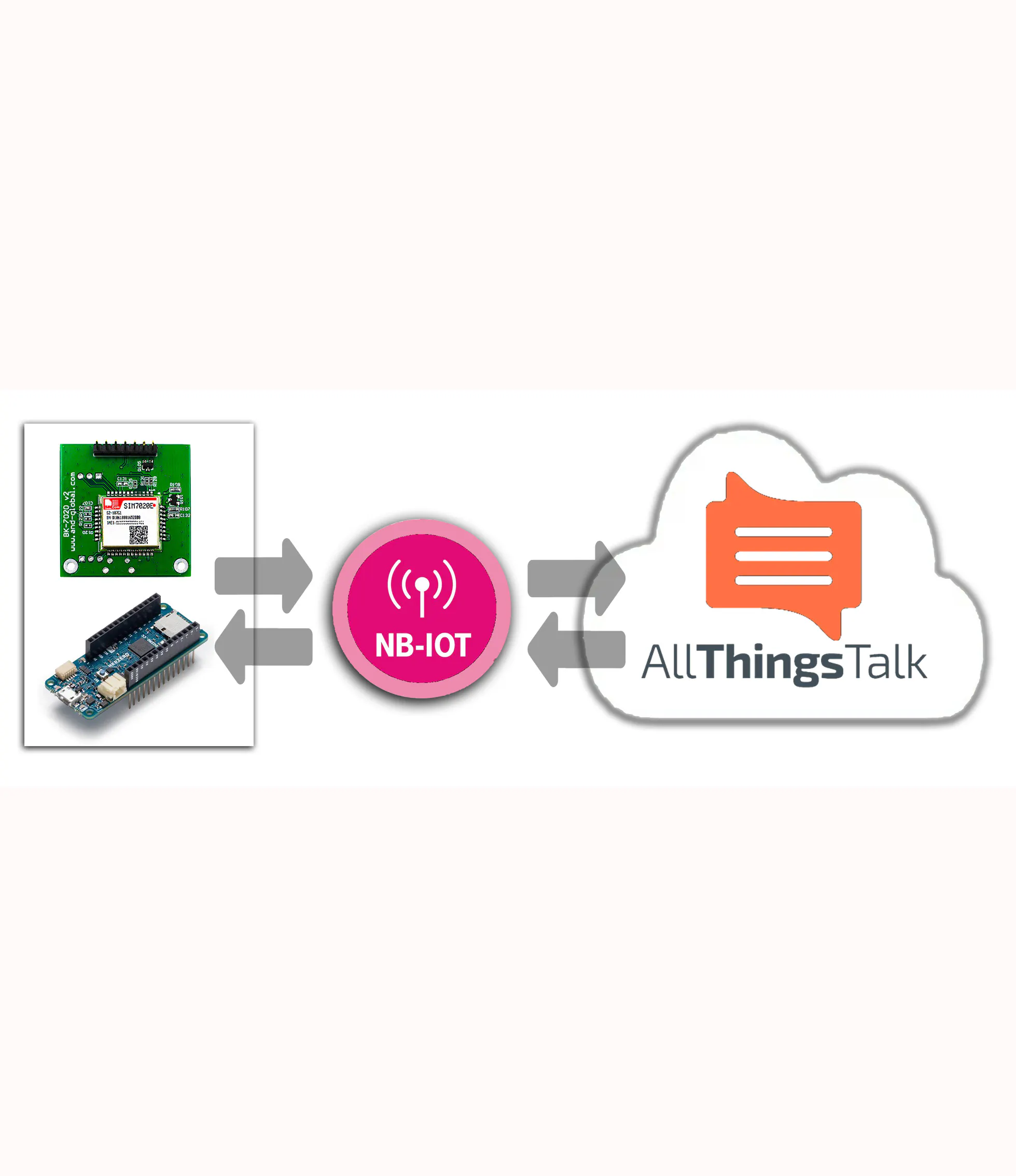Published

# Arduino NB-IoT with SIM7020 and T-Mobile

Demonstration program on how to drive a SIM7020 NB-IoT modem, then set up a dashboard using T-Mobile's network and AllThingsTalk API.

IntermediateWork in progress2 hours6,744## Things used in this project

### Hardware componentsArduino MKR Zero
×1
 BK-SIM7020E
×1

### Software apps and online servicesArduino IDEAllThingsTalk Maker
 T-Mobile NBiot

## Schematics

### Arduino - BK-SIM7020E Connections

Arduino - BK-SIM7020E Connections## Code

### SIM7020zero.ino

C/C++
sim7020 Demo Sketch
```#include "SIM7020_script.h"
//
// Sim7020E demo to AllThingsTalk Cloud via Nbiot T-Mobile
// March 2019 V 1.00
// J.Vos - Voske
//
//
//
// Pinning to Module :
// MKR pin13 = RX = PB23_S5_RX => SerCom5 PAD3
// MKR pin14 = TX = PB22_S5_TX => SerCom5 PAD2
// MKR Pin6 = SLEEP
// MKR Pin7= PWR

#define TIMEOUT 200        // AT-command timeout in 100ms  -> 200=20sec
#define COMMANDMAX 1024
const int SleepPin = 6;
const int PowerPin = 7;
char SimError[] = "**Error ";
char SimTimeout[] = "**Timeout ";
char MyImei;
char MyIp;
char MyMfr;
char MyCicc;
char MyModel;
int TimeOut=TIMEOUT;

char simresult[COMMANDMAX];  // AT command result buffer

void setup(){
pinMode(SleepPin,OUTPUT);
pinMode(PowerPin,OUTPUT);
digitalWrite(SleepPin,LOW); // let DTR in pull-UP
digitalWrite(PowerPin,HIGH); // Keep PowerKey high

int t=10;  //Initialize serial and wait for port to open, max 10 second waiting
Serial.begin(921600UL);
while (!Serial) {
; delay(1000);
if ( (t--)== 0 ) break;
}

Serial.print("SIM Demo starts.");
Powercycle();   // run power cycle
Serial1.begin(115200); // RX/TX serial connect SIM7020

}

void loop() {
delay(1000);
//SerialCheck();

//Serial.print("\n** Imei:");Serial.println(MyImei);
//Serial.print("** cICC:");Serial.println(MyCicc);
//Serial.print("** Mfr: ");Serial.println(MyMfr);
//Serial.print("** Model: ");Serial.println(MyModel);
delay(2000);

if  (runscript(NBstart)!=1 ) transparantmode();
delay(1000);
if  (runscript(NBopensocket)!=1 ) transparantmode();
delay(1000);
while (1)
{
delay(60000); // wait 1 MINUTE //
}

}

// send payload 2 integers in hex format (2x4 = 8 characters) - MIXED UP NIBBLES FOR DEMO !!!
{
char c=0;
int t=0,n=0;
simresult=0;
Serial1.flush();
Serial1.print("AT+CSOSEND=0,8,\"");
Serial1.print( ((byte) value0)&0x0F,HEX);          // setup every nibble (4bits) individual, as print-HEX formatting does not support leading Zero
Serial1.print( ((byte) value0>>4)&0x0F,HEX);       // mixing up lower and higher nibbles to create bigger random number
Serial1.print( ((byte) value0>>8)&0x0F,HEX);
Serial1.print( ((byte) value0>>12)&0x0F,HEX);
Serial1.print( ((byte) value1)&0x0F,HEX);
Serial1.print( ((byte) value1>>12)&0x0F,HEX);
Serial1.print( ((byte) value1>>4)&0x0F,HEX);
Serial1.print( ((byte) value1>>8)&0x0F,HEX);
Serial1.print('\"');
Serial1.print('\r');Serial1.print('\n');
while ( n<=TimeOut ){
if (Serial1.available()) {
simresult[t]=c;simresult[++t]=0;
Serial.write(c);
}
else {delay(100);n++;};
if ( (t>3) &&  (  ( (simresult[t-4]=='O')&&(simresult[t-3]=='K')&&(simresult[t-2]=='\r')&&(simresult[t-1]=='\n') )  ||  (  (simresult[t-4]=='O')&&(simresult[t-3]=='R')&&(simresult[t-2]=='\r')&&(simresult[t-1]=='\n')  )  ) )  break;
}
if (n>=TimeOut) {Serial.println(SimTimeout); return(-1);}
else { if( (simresult[t-4]=='O')&&(simresult[t-3]=='R') ) {Serial.println(SimError);return(0);}
else return(1);}
}

long int SerialCheck()
{
int n;
int t;
int b=0;
TimeOut =3;
Serial.print("\n** Serial1 Test:");
Serial1.end(); delay(1000);
for (t=0;t<BAUDRATES;++t){
Serial.print("\nBd=");Serial.println(Baudrates[t]);
Serial1.begin((long int) Baudrates[t]);
delay(1000);
if (runscript(NBat)!=-1) b=t; // send AT command if baudrate works, then remeber baudrate
for (n=0;n<24;++n) transparantmode();delay(200); // read responses
Serial1.end(); delay(1000);
}
Serial.print("\n** Serial1 Test Max Bd=");Serial.print((long int) Baudrates[b]);
Serial1.begin((long int) Baudrates[b]);
TimeOut=TIMEOUT;
return((long int) Baudrates[b]); // return highest speed
}

// run script -> array of strings ending with 0-string , result 1=ok, 0= error -1=timeout
int runscript(const char** scrpt){
int t=0;
int s=1;
//Serial.println("\n** SimScript:");
while ( strlen( scrpt[t]) > 1){
s = writecommand(scrpt[t]);
delay(1000);
if ( s==1 ) ++t;
else break;
}
return(s);
}

// write one commandline to Serial, result 1=ok, 0= error -1=timeout
int writecommand(const char* cmd){
char c=0;
int t=0,n=0;
simresult=0;
Serial1.flush();
Serial1.print(cmd);Serial1.print('\r');Serial1.print('\n');
while ( n<=TimeOut ){
if (Serial1.available()) {
simresult[t]=c;simresult[++t]=0;
Serial.write(c);
}
else {delay(100);n++;};
if ( (t>3) &&  (  ( (simresult[t-4]=='O')&&(simresult[t-3]=='K')&&(simresult[t-2]=='\r')&&(simresult[t-1]=='\n') )  ||  (  (simresult[t-4]=='O')&&(simresult[t-3]=='R')&&(simresult[t-2]=='\r')&&(simresult[t-1]=='\n')  )  ) )  break;
}
if (n>=TimeOut) {Serial.println(SimTimeout); return(-1);}
else { if( (simresult[t-4]=='O')&&(simresult[t-3]=='R') ) {Serial.println(SimError);return(0);}
else return(1);}
}

// transparant mode
void transparantmode() {
char c1=0,c2=0;
Serial.println("*Transparant AT-mode - type *X to eXit -");
while(1)
{
if (Serial.available()) {      // If anything comes in Serial (USB),
c2=c1;
if ( (c1=='X') && (c2=='*') ) break;
else
Serial1.write(c1);   // read it and send it out Serial1
}

if (Serial1.available()) {     // If anything comes in Serial1
}
}
Serial1.write(c1);
while ( Serial.available() ) {c1=Serial.read(); Serial1.write(c1);} // flush USB write buffer

}

void Powercycle()
{
Serial.print("\n**PowerCycle.");
digitalWrite(PowerPin,HIGH);
Serial.print(".high");
delay(2000);
digitalWrite(PowerPin,LOW);
Serial.print("..low");
delay(2000);
digitalWrite(PowerPin,HIGH);
Serial.print("..high\n");
//digitalWrite(SleepPin,HIGH);
delay(2000);
}

void Poweroff()
{
Serial.print("\n**Power..");
digitalWrite(PowerPin,LOW); // let DTR pull up
delay(3000);
Serial.print(".off");
}

// read iMei and put in global variable,  returns status -1,0,1
int t=0,n=0,s=0;
s=runscript(NBgetimei);
if (s!=1) return(s);
else {
// anlyse result in simresult buffer
t=0;n=0;
while(simresult[t++] !='\n');
while(simresult[t] !='\n') MyImei[n++]=simresult[t++]; // copy second line
MyImei[n]=0;
return (s);
}
} // end function

// read Mfr and put in global variable,  returns status -1,0,1
int t=0,n=0,s=0;
s=runscript(NBgetmfr);
if (s!=1) return(s);
else {
// anlyse result in simresult buffer
t=0;n=0;
while(simresult[t++] !='\n');
while(simresult[t] !='\n') MyMfr[n++]=simresult[t++]; // copy second line
MyMfr[n]=0;
return (s);
}
} // end function

// read CICC and put in global variable, returns status -1,0,1
int t=0,n=0,s=0;
s=runscript(NBgetcicc);
if (s!=1) return(s);
else {
// anlyse result in simresult buffer
t=0;n=0;
while(simresult[t++] !='\n');
while(simresult[t] !='\n') MyCicc[n++]=simresult[t++]; // copy second line
MyCicc[n]=0;
return (s);
}
} // end function

// read Model and put in global variable, r returns status -1,0,1
int t=0,n=0,s=0;
s=runscript(NBgetmodel);
if (s!=1) return(s);
else {
// anlyse result in simresult buffer
t=0;n=0;
while(simresult[t++] !='\n');
while(simresult[t] !='\n') MyModel[n++]=simresult[t++]; // copy second line
MyModel[n]=0;
return (s);
}
} // end function
```

### Sim7020 Demo Include file

C/C++
Include file with AT-Scripts
```#define BAUDRATES 15
const long int Baudrates[BAUDRATES] = {0,110,300,1200,2400,4800,9600,19200,38400,57600,115200,230400,460800,921600,3000000};

const char* NBstart[] = {
"ATZ",
"AT+CFUN=0",
"AT+CREG=2",
"AT*MCGDEFCONT=\"IP\",\"cdp.iot.t-mobile.nl\"",
"AT+CFUN=1",
"AT+CBAND=8",
"AT+CGCONTRDP",
"AT+CSQ",
"\0"  // end script with 0x00
};

const char* NBopensocket[] = {
"AT+CSOC=1,2,1",
"AT+CSOCON=0,15683,\"172.27.131.100\"", // T-Mobile Server socket 0
"\0"     // end script with 0x00
};

const char* NBclosesocket[] = {
"AT+CSODIS=0",
"AT+CSOCL=0",
"AT+CGACT=0,1",
"\0"     // end script with 0x00
};

const char* NBhelloworld[] = {
"AT+CSOSEND=0,0,\"Hello World!\"",
"\0"     // end script with 0x00
};

const char* NBgetimei[] = {
"AT+GSN",
"\0"     // end script with  0x00
};

const char* NBgetmfr[] = {
"AT+GMI",
"\0"     // end script with  0x00
};

const char* NBgetcicc[] = {
"AT+CCID",
"\0"     // end script with  0x00
};

const char* NBgetmodel[] = {
"AT+GMM",
"\0"     // end script with  0x00
};

const char* NBdefault[] = {
"ATZ",
"\0"     // end script with  0x00
};

const char* NBat[] = {
"AT",
"\0"     // end script with  0x00
};

const char* NBss921600[] = {
"AT+IPR=921600",
"\0"     // end script with  0x00
};

const char* NBss0[] = {
"AT+IPR=0",
"\0"     // end script with  0x00
};
```

## Credits

### JayV

12 projects • 21 followers